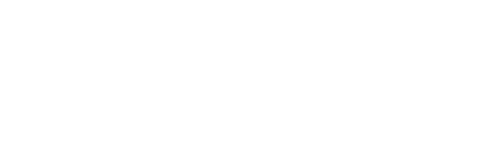# Deletion at the start, middle and end of the LinkedList in a single program in C++

Deletion at the start, middle, and end of the LinkedList in a single program in C++.

Linked list operations covered in the program

1. Deletion at the start of the LinkedList.
2. Deletion in the middle Of the LinkedList.
3. Deletion at the end of the LinkedList.
```#include <iostream>
using namespace std;

struct Node {
int data;
struct Node* next;
};

// count total nodes
{
int count = 0;
count++;
}
return count;
}
// Deletes the middle node and returns  the head of the list after modifications.
{
return NULL;
return NULL;
}

// Find the total nodes in the list

// Find the middle node in the list
int mid = count / 2;

// Delete the middle node in the list
while (mid-- > 1) {
}
// Delete the middle node in the list
}

void printList(struct Node* ptr)
{
while (ptr != NULL) {
cout << ptr->data << "->";
ptr = ptr->next;
}
cout << "NULL\n";
}
//deleting the first node in the list
{
return NULL;

// Move the head pointer to the next node in the list
delete tempNode;
}
//delete last node from the list
{
return NULL;

return NULL;
}
// first find the second last node in the list
while (second_last->next->next != NULL)
second_last = second_last->next;

// Deleting the last node in the list
delete (second_last->next);

// set next of second_last node to null in the list
second_last->next = NULL;

}

void push(struct Node** head, int new_data)
{
struct Node* newNode = new Node;
newNode->data = new_data;
}
int main()
{
// creating  the linked list by pushing the data.
Node* temp;
for (temp = head; temp != NULL; temp = temp->next)
cout << temp->data << ":";
if(temp == NULL)
cout<<"NULL"<<endl;
//delete first node in the list
for (temp = head; temp != NULL; temp = temp->next)
cout << temp->data << ":";
if(temp == NULL)
cout<<"NULL"<<endl;
//delete last node in the list
cout<<"Linked list Demo after deleting last node"<<endl;
for (temp = head; temp != NULL; temp = temp->next)
cout << temp->data << ":";
if(temp == NULL)
cout<<"NULL"<<endl;

cout << "Demo of Linked List after deletion  of the middle node."<<endl;
Cout<<"Good by T4Tutorials.com"<<endl;
return 0;
}
```

OutputExcercise

delete the last node in linked list C++.
delete all nodes in linked list C++.
delete the first node in linked list C++.
delete the last node in linked list C++.
insertion and deletion in a linked list in C++ program.
remove elements from linked list C++.
delete the last node in the linked list in C++.
delete a node from the linked list algorithm C++.
delete the smallest node linked list C++.